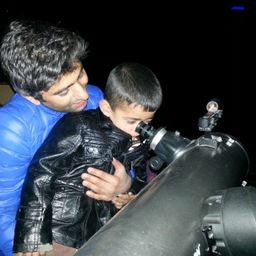Gravitation

### Discussion

You must be signed in to discuss.
##### Top Physics 101 Mechanics EducatorsLB##### Aspen F.

University of Sheffield

Lectures

Join Bootcamp

### Video Transcript

we know the total energy, um, is equal to. In fact, the square is equal to ah, be time. See whole square bliss. Ah, rest more synergy. Uh, square on and we have yeah, is equal to five times rest More synergy. And therefore we have five times rest More synergy. All square is equal to ah b times C hold square unless, huh rest mass Energy square on this imply stage. Uh, be time. See, Old square is equal to 24. Multiply by e r. Hold scape. No, let's sold for B be. His momentum is equal to screen route off 24 uh, multiply Hi Rist Maas Not sorry. Wrist monster Energy divide by speed of light. Well, let's plug into values be equals. Uh, scared of 24 Multiply by. Well, rest mass energy is 0.511 million electron volts divided by. See And this gives us, um 2.50 1,000,000 electron world divided by speed. Off light on, right? Um no, it's do part the second part. Well, part b well again using, um the same relation we have. Ah b is equal to the square root of 24 times. Um wrist Masanori Gene Divided by sea No. In this case, rest most energy is 9 38 13 million electron volts. Therefore, be physical. To scrape it off 24 multiply by 9 38.3 a 1,000,000 electron Walt, divided by see And this gives us 4600 1,000,000 electron world divided by speed of light.#### Topics

Gravitation

##### Top Physics 101 Mechanics EducatorsLB##### Aspen F.

University of Sheffield

Lectures

Join Bootcamp